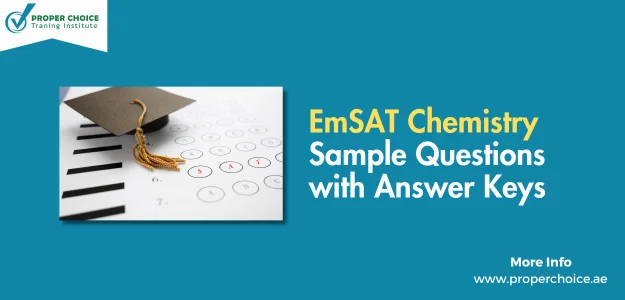# EmSAT Chemistry Sample Questions with Answer Keys – 2023Looking for EmSAT Chemistry sample questions with answer keys for 2023? Explore our comprehensive collection to boost your preparation. Practice with confidence and ace your EmSAT Chemistry exam. Get started today!

Question 1

30 mL of 2.0 M HCL and 25.0 mL of 1.5 M NaOH are mixed together in a Styrofoam cup and the reaction below occurs

H+ + OHH2O(l)

The temperature of the reaction rises from 25.00to 32.16over the course of the reaction. Assuming the density of the solution is 1.0 g/mL and the specific heat of the mixture is 4.18 J/g, calculate the enthalpy of the reaction.

• A) 1646.08 J
• B) 1970.03 J
• C) 2173.55 J
• D) 1892.79 J

Question 2

The oxidation state of Sulfur in H2SO4 is?

• A) 2
• B) 4
• C) 6
• D) – 8

Question 3

Calculate the pH of 0.20 molar solution of HC2H3O2, with Ka = 1.810-5

• A) 1.9
• B) 2.7
• C) 3.3
• D) 4.0

Question 4

Is the reaction below thermodynamically favored at 25℃ °⁄

$CO\left(g\right)+H_2O\left(g\right)\ →\ CO_2\left(g\right)+H_2\left(g\right)\ ΔH°=-41.2\ kj⁄mol\ \ ΔS°\ =\ 135\ j⁄mol\ .\ K$

• A) Reaction is thermodynamically favored at 25°C
• B) Reaction is thermodynamically unfavored at 25°C
• C) Reaction is at equilibrium at 25°C
• D) Cannot predict favorability for the reaction

Questions 5

pH value of 0.010 M HCl solution is

• A) 0
• B) 1
• C) 2
• D) 3

Question 6

$Fe+2\ HCI\ →\ FeCI_2+H_2$

In the chemical equation above, which of the following element got oxidized?

• A) H
• B) Cl
• C) Fe
• D) FeCl2

Question 7

When a copper bar is submerged in a solution of silver nitrate, the silver ions reduced to solid silver and the copper bar will be oxidized into copper ions. Which of the following represents a balanced redox reaction?

• A) Ag+ + eAg(s)
• B) Cu(s)Cu2+ + 2e
• C) Ag+ + Cu(s)Ag(s) + Cu2+
• D) 2Ag+ + Cu(s)2Ag(s) + Cu2+

Question 8

$Fe+2\ HCI\ →\ FeCI_2+H_2$

In the chemical equation above, which of the following is reducing agent?

• A) Fe
• B) HCl
• C) H2
• D) FeCl2

Question 9

Redox reaction occurs between

•  A) two metals
• B) two non – metals
• C) metals and non–metals
• D) Transitional metal

Question 10

Oxidation state of Alkali Earth Metals is

• A) + 1
• B) + 2
• C) +3
• D) – 2